August 25, 2012

# Heap datastructure in pictures

A binary heap (generally referred as heap) is a rooted left-complete binary tree which has two properties

1)	heap property

2)	shape property


Wow. Now, that is a lot of jargon. Let's see what each word in the definition means.

What is a tree?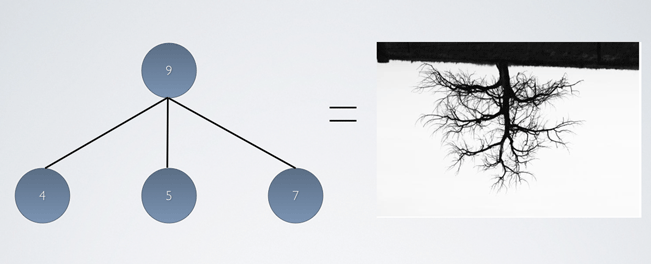A tree is just a set of nodes connected by edges. Yikes !

Let's put this in a picture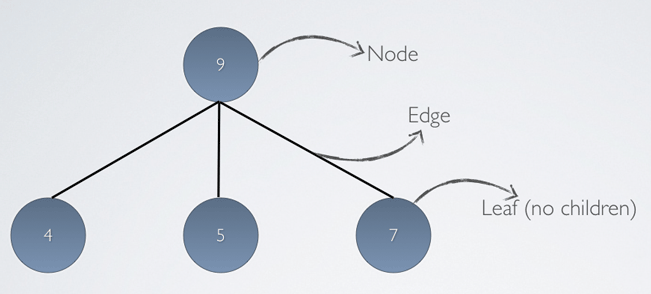What is a binary tree?

A binary tree is simply a tree with utmost 2 nodes.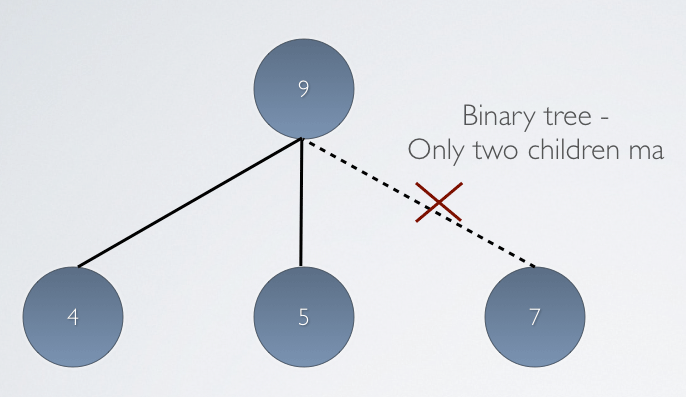What is a rooted binary tree?

A root is just a node which is specially qualified as root. For clarity, a root is generally drawn as the topmost node when graphically representing the datastructure.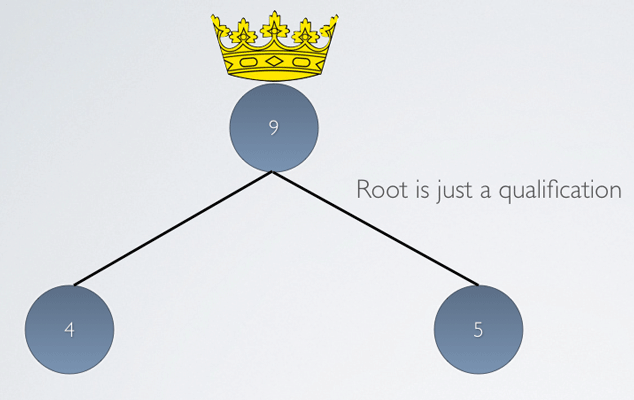What is a left-complete binary tree?

When all the levels of the binary tree has nodes except the last level, then it is a complete binary tree.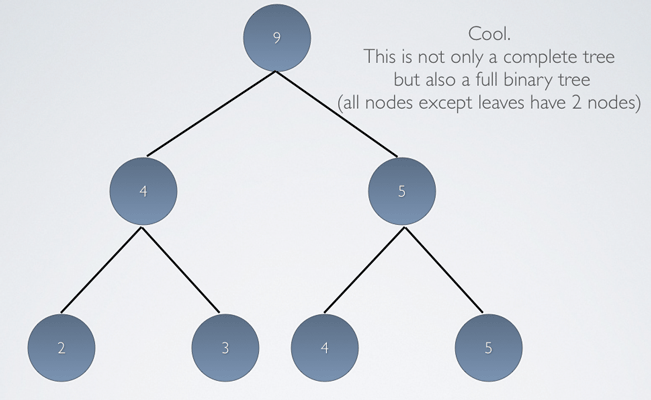Even if the last one does not have all the nodes, then it should be filled in such a way that the left most nodes are filled before the right ones. ie. There should not be any gaps in the middle. Now, that makes a left-complete binary tree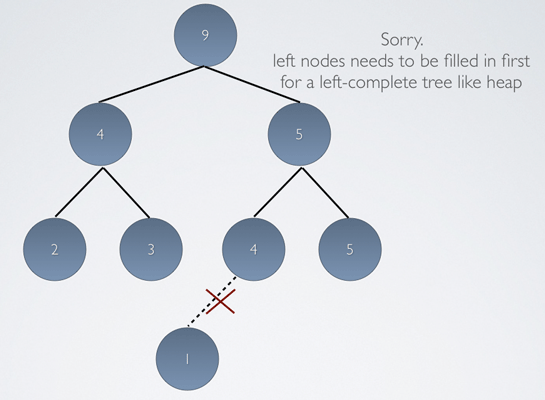A legal heap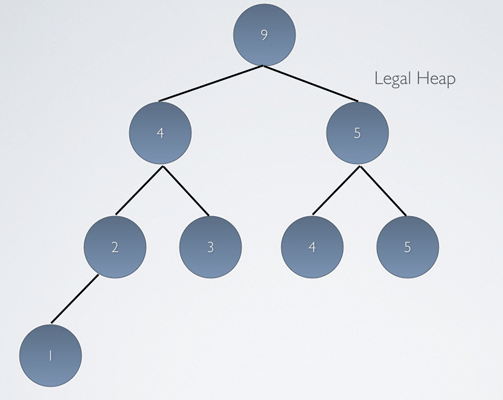Now that clears up "A binary heap (generally referred as heap) is a rooted complete binary tree".

Let's see what heap and the shape properties mean.

Shape-property :

Well, I cheated. The shape property of a heap simply says that the heap must be a left-complete binary tree. So, dangling nodes can appear only on the left-side. No gaps in between.

Heap property :

The children/child of each node should be lesser than the node itself (in case of max heap)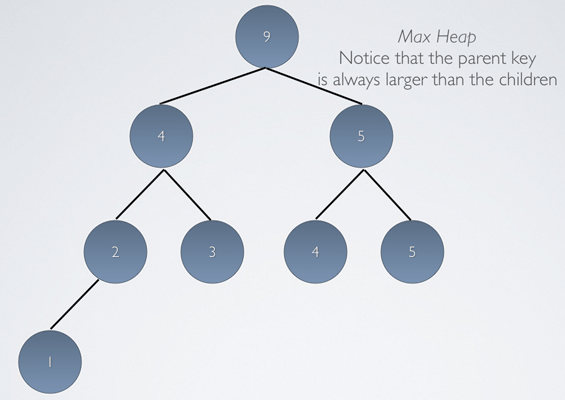The children/child of each node should be greater than the node itself (in case of min heap)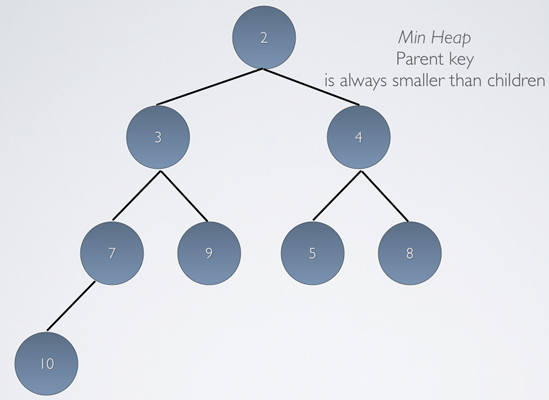Representing Heap in an array :

The easiest and the general way of representing a heap is using an array. You'll see how the complete/shape property of the heap makes it easier to represent in an array.

Just read the tree left to right (level-order traversal, if you like it that way)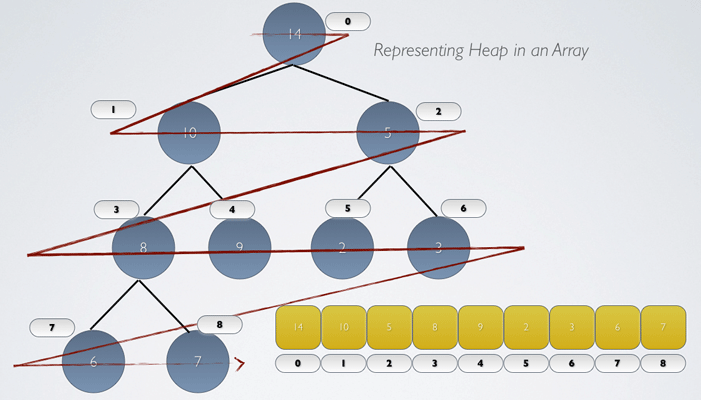Calculating location of children and parent in array :

The root is the first item of the array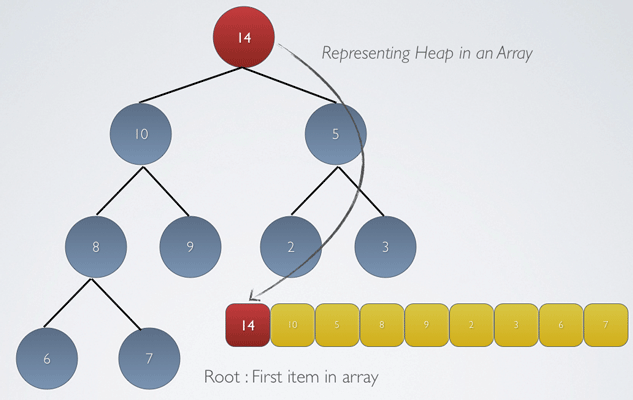Indices of Children can be calculated, known a parent index, using the simple calculation :

Child 1 = 2i +1
Child 2 = 2i +2

where i is the index of the parent


Again, the index of the parent can be calculated by reversing the formula

Index of parent given a child index is

Parent = i/2  (round the result to the lower bound)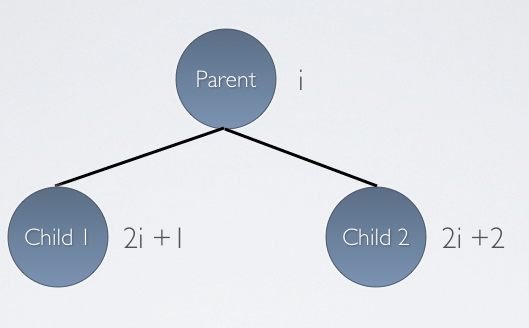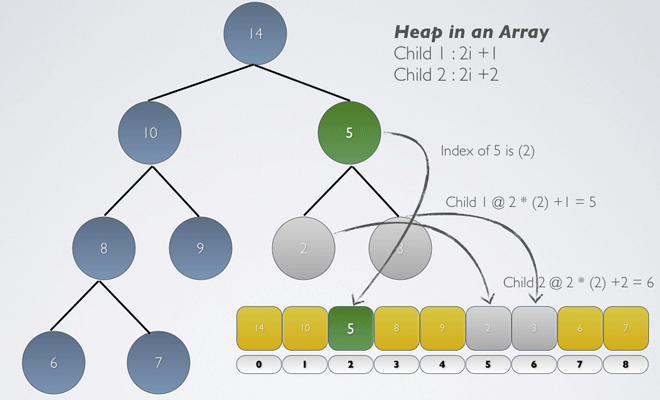So, considering the image above, the children of 5 at index 2 are items located in indices 5 and 6 which are 2 and 3

Fun facts :

1) A sorted array is a min heap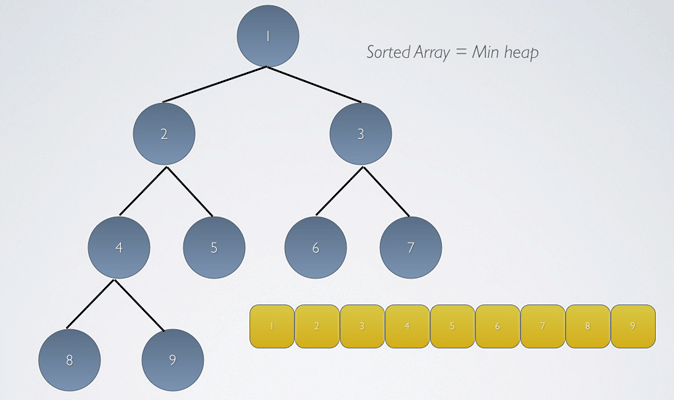2) Unlike a Stack, a JVM heap has nothing to do with the heap data structure. Though rumor has it that the usage of name "heap" for its memory area comes from one of the early languages using Heap datastructure to store objects in memory at runtime.

1 million nodes = 20 levels :

Since Heap is a complete binary tree (remember : no spaces in between), the maximum number of levels is easy to calculate.

First level 	=	1

Second level 	=	2 	=	2^1

Third level 	=	4 	= 	2^2

Fourth level 	= 	8	=	2^3


...

eg. until 4th level, there are 15 nodes.

or

total number of nodes until 4th level = 2^4 -1.


In other words, the height of the heap = log base 2 (n) (round to the upper bound)

Applications of Heaps:

A heap could be used to implement a priority queue, which is a queue that orders entities not a on first-come first-serve basis, but on a priority basis. So, since the heap has the minimum or the maximum key at the top, the next "priority" item is at the top with a removal time of 0(1).

Also, Heaps are used in the Heap Sorting algorithm which has the worst case performance of 0 (n log n). No wonder Heap sort is the preferred sorting algorithm for all the mission critical applications.